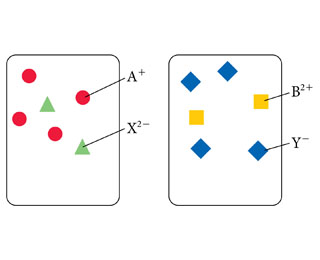# Problem: Consider the generic ionic compounds with the formulas A2X and BY2 and the following solubility rules:A2X soluble; BY2 soluble; AY insoluble; BX soluble.Assume A+ ions are circles, B2+ ions are squares, X2- ions are trianges, and Y- ions are diamonds. Solutions of the two compounds (A2X and BY2) can be represented as follows:Draw a molecular level representation showing the result of mixing the two solutions given.

###### FREE Expert Solution

We have to draw a molecular level representation of the reaction between A2X and BY2that produces AY and BX.

A2X + BY2→ 2 AY + BX

We have been given the information that AY is an insoluble compound while BX is soluble.

We know that soluble ionic compounds are present as their ions in the solution.

89% (136 ratings)###### Problem Details

Consider the generic ionic compounds with the formulas A2X and BY2 and the following solubility rules:
A2X soluble; BY2 soluble; AY insoluble; BX soluble.
Assume A+ ions are circles, B2+ ions are squares, X2- ions are trianges, and Y- ions are diamonds. Solutions of the two compounds (A2X and BY2) can be represented as follows:Draw a molecular level representation showing the result of mixing the two solutions given.

Frequently Asked Questions

What scientific concept do you need to know in order to solve this problem?

Our tutors have indicated that to solve this problem you will need to apply the Molecular Equations concept. You can view video lessons to learn Molecular Equations. Or if you need more Molecular Equations practice, you can also practice Molecular Equations practice problems.

What professor is this problem relevant for?

Based on our data, we think this problem is relevant for Professor Blankenship's class at UGA.